Next: 4.4.2 Boundary Models Up: 4.4 Assembling of the Previous: 4.4 Assembling of the

## 4.4.1 Volume Models

A lot of partial differential equations can be discretized so that finally a sparse matrix results which represents a linear equation system that has to be solved (Fig. 4.9). In case of non linearity an iteration is necessary where successive approximations are calculated. The condition for the nonlinear system

 f (xs) = 0 (4.6)

is satisfied in case of
 lim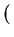xi= xs (4.7)

Looking at the first order of the Taylor series

 f (xs) = f (xi) +.xs - xi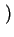+ Oxs - xi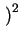= 0 (4.8)

it is obvious that in case of a linear approximation where terms of higher order are neglected ( Oxs - xi= 0) the resulting linear equation system
 F(xi) .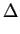xi + f (xi) = R (4.9)

satisfies the postulated condition when R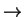0. In case of linear systems the solution can immediately be determined by
 xi + 1 = xi +xi = xs (4.10)

The vector f(x) denotes the so called Residual function

 f (x) =(4.11)

which is a vector of functions of the searched quantities q1, q2, ... , qn. Its derivative stands for the system matrix or also called Jacobian Matrix and can be calculated using the following rule
 F(x) =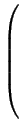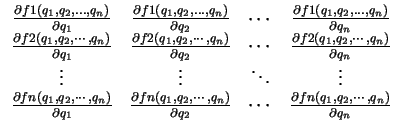(4.12)

AMIGOS uses this mathematical expression profitably so that an automatic assembling of the global stiffness matrix is done depending on the basis of a discretized simulation domain. It simplifies the task of global assembling of all grid points and reduces it to a local process where the PDE system has to be discretized only on a single element. To preserve one's survey it is much easier to handle just the smaller local part of the discretization than dealing with the global assembling step (Fig. 4.9). Furthermore, AMIGOS releases the model developer from the duty to calculate the Jacobian matrix using its derivative operator that derives the residual function symbolically.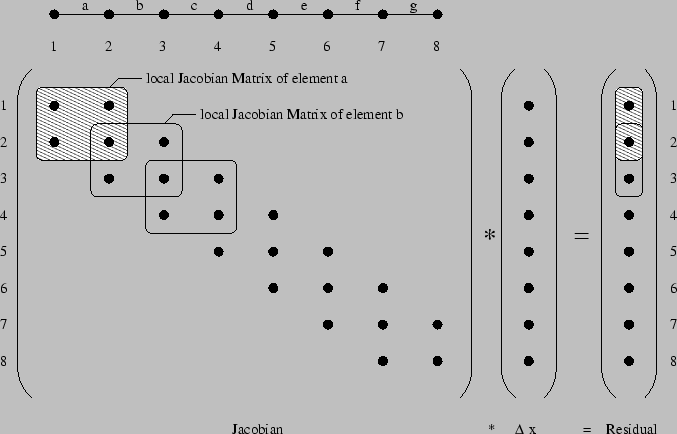Next: 4.4.2 Boundary Models Up: 4.4 Assembling of the Previous: 4.4 Assembling of the